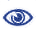# Prague Stringology Conference 2015

Efficient Algorithm for δ-Approximate Jumbled Pattern Matching

 Abstract: The Jumbled Pattern Matching problem consists on finding substrings which can be permuted to be equal to a given pattern. Similarly the δ-Approximate Jumbled Pattern Matching problem asks for substrings equivalent to a permutation of the given pattern, but allowing a vector of possible errors δ. Here we provide a new efficient solution for the δ-Approximate Jumbled Pattern Matching problem using indexing tables and bit vectors which, according to the experimental results, gives a speed up about 1.5–3.5 times faster than the solution based on Wavelet trees. This speed up depends mainly of the size of the alphabet. Further there are presented some solutions to another problems related to δ-Approximate Jumbled Pattern Matching, as the All Matching problem, where it is necessary to calculate all the occurrences of a given pattern allowing an error in the text, or the Min-Error problem, where the objective is to find the occurrences which are closer to the pattern.

 Download paper:PostScript PDF BibTeX reference
 Download presentation: